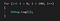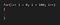Objective: Understand what a for loop is and how to implement.

Loops are functions used to repeat actions. If, for example, you want to increment a variable once every three seconds you would use a loop.

A for Loop looks like this

For this tutorial we are going to use this for loop to print out numbers 1–100.The debug log will print out the value of the “i” on the console view

Now let’s break this down. Inside the parentheses is how long the loop repeats using an int variable.

This lets us know we are starting the loop and keeping track of how many times the loop repeats.

The “i” represents the variable. Standard practice is Csharp is to use “i” however you could label it however you see fit.

Next in the parentheses is to set the max limit to increment to. For the sake of this tutorial lets set the max value at 100.

If “i” is less than the max value (100) the loop will repeat itself. Every time this code cycles we want to increment the value of “i”.This will increase the value of “i” by one every cycle.

This with the debug log command the console view will have 0–100 printed out. If we wanted to do only the even numbers, we need to take a few more steps.

We need to use an if statement and divide the value by 2, if there is a remainder then it is an odd number.

This will print out only the even values, you could switch to odds by switching the if statement to ==1.

If you want to only display a specific number, you set the variable to equal the set number.

That’s it, this is how you set up a for loop. You can use for loops in your game to shatter an object, which requires looping through each individual piece and adding physics. You can use for loops for many different occasions in your game, experiment with your code and I’ll see you in the next tutorial.